#Function Repository Resource:

# AntColonyOptimization

Solve a combinatorial optimization problem using an Ant Colony Optimization (ACO) algorithm

Contributed by: Lars Ulke-Winter
 ResourceFunction["AntColonyOptimization"][array,fitness] returns the minimum found result for the function fitness with possible values for each variable given in array. ResourceFunction["AntColonyOptimization"][array,fitness,All] returns the intermediate optimization step results and optimization parameters as a summary Association.

## Details and Options

ResourceFunction["AntColonyOptimization"] is a global stochastic optimization method for the solution of combinatorial arrangement problems. It imitates the swarm communication behaviour of ants during foraging (Stigmergic Optimization).
The optimization is based on the exchange of information between individuals (randomly generated solutions) using a virtual pheromone trail for the information exchange.
AntColonyOptimization finds a solution of vector x, where the ith element of x is chosen from the values of the ith list in array, such that fitness[x] has the smallest positive value found.
The input array takes a list of lists with available values at each row. The lists need not have uniform length.
The fitness function to be minimized must return a positive scalar value. Therefore, the standard method of negating the objective function to convert a minimization problem into a maximization problem doesn’t work in this ACO implementation.
Limitation: This implementation does not consider dependencies of design variables among each other. The assignment of the design variables must be independent of the settings of the others. The definition of the search space is therefore possible using only a given array and does therefore not require a given tree or graph structure of the search space. Optimization problems such as the traveling salesman cannot be encoded in this way.
Optimization parameters given as options include:
 "PopulationSize" 500 number of ants "MaxIterations" 200 maximum iterations "FinishAtPheromoneThreshold" 0.95 stop criterion "PheromoneFunction" (1/#) & pheromone concentration to be deposited depending on fitness "WeatheringFactor" 0.05 fraction of decreasing pheromone concentration (selection probability) per iteration "ProbabilitySelectBestPath" 0.02 proportion of generated solution alternatives that currently prefer the best solution
The computation will stop if the selection probability for a variable state exceeds the value of "FinishAtPheromoneThreshold".
"PheromoneFunction" is a function whose result marks the selection probability for the next step. By default, larger fitness results receive lower marking values than smaller fitness results.
Optimization parameters control the runtime behavior and solution quality as a tradeoff between exploitation and exploration. These can be modified depending on the target function and the number of variables, e.g. by trial and error.
The option "ProbabilitySelectBestPath" takes values between zero and one. Smaller values use probabilistic selecting resulting in slower convergence but exploring more possibilities.
The current minimal selection probability for a variable state is monitored during the iterations. The smaller it is, the greater the ability to explore new solutions.
The output of ResourceFunction["AntColonyOptimization"] is a List representing the best found solution. Due to the stochastic search method, this optimum does not necessarily have to be global, thus better solutions may exist.
When the third argument is All the output is an Association with keys:
 "Best" variable allocation which minimizes fitness "Iterations" for each iteration step: {arguments which minimize fitness, normalized pheromone matrix, and current minimal selection probability for a variable state} "NumberOfFunctionCalls" number of calls made to the fitness calls

## Examples

### Basic Examples

Create an array of possible assignments:

 In:=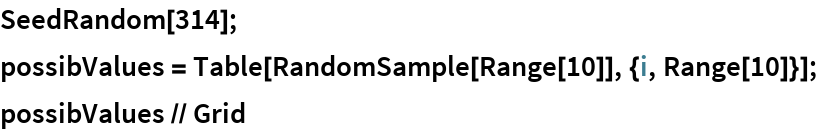Out=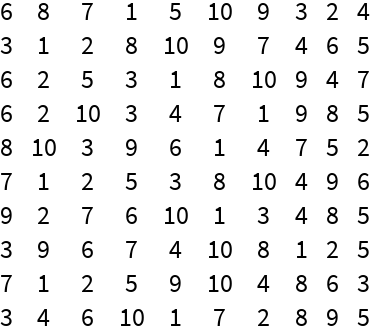Define an objective function to minimize:

 In:=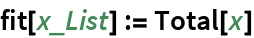Search for minimum:

 In:=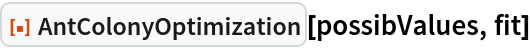Out=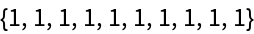Return the complete iteration process using the third argument of All:

 In:=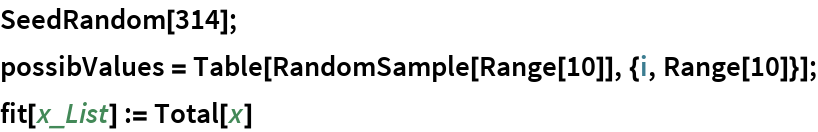In:=Out=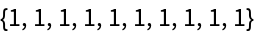Convergence behavior:

 In:=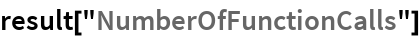Out=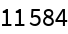In:=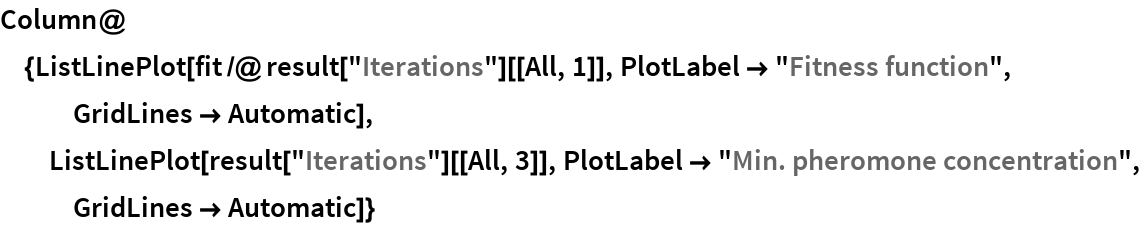Out=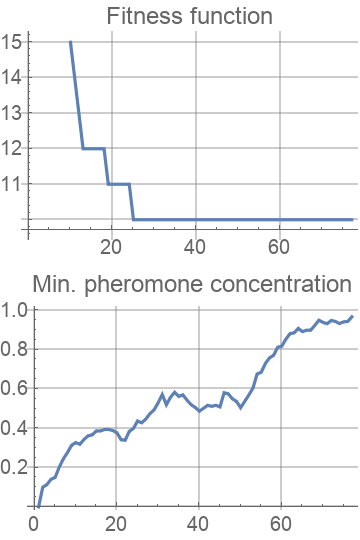In:=Out=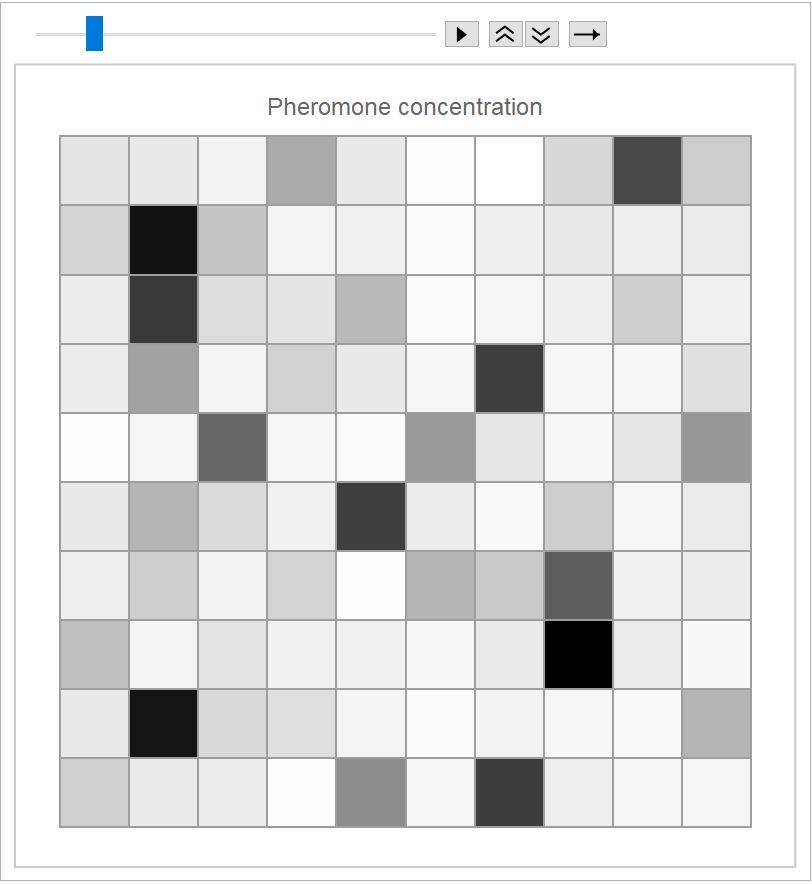### Options

The above example with default options shown :

 In:=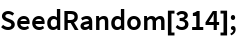In:=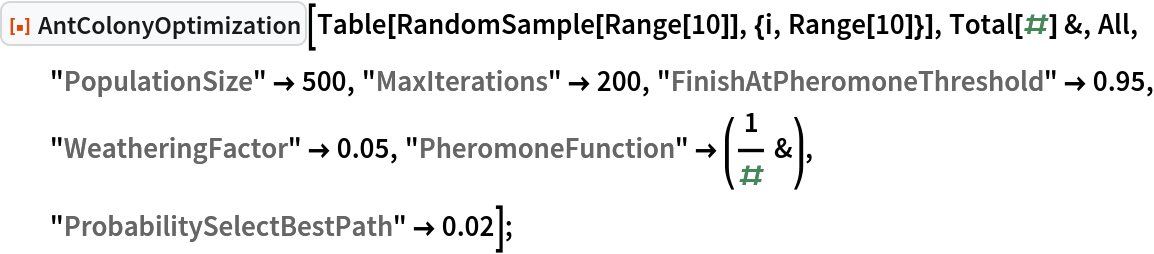In:=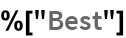Out=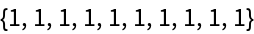In:=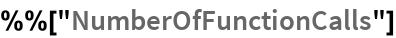Out=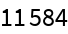#### FinishAtPheromoneThreshold

Terminate if a quarter of individuals have the same fitness value:

 In:=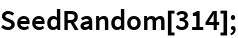In:=In:=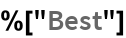Out=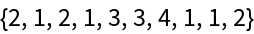In:=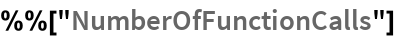Out=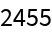#### ProbabilitySelectBestPath

Have 50% of the individuals select the current shortest path (low exploration):

 In:=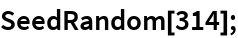In:=In:=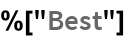Out=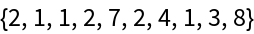In:=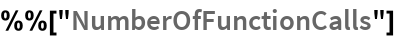Out=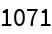#### PheromoneFunction

Use a lower pheromone marking at each step depending on individual fitness value (low convergence speed, high exploration):

 In:=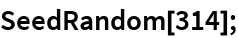In:=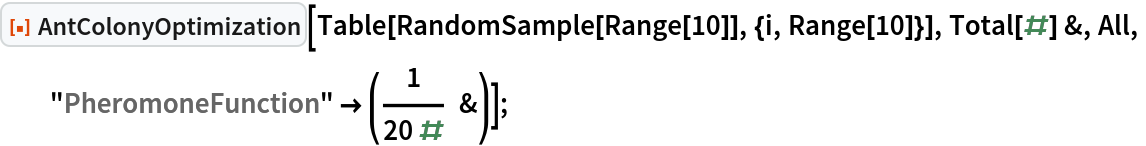In:=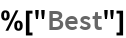Out=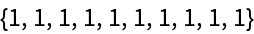In:=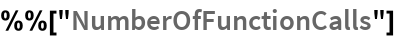Out=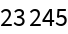#### WeatheringFactor

Reduce the selection probability for less-used paths more slowly:

 In:=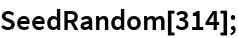In:=In:=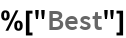Out=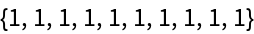In:=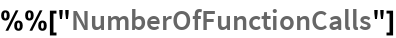Out=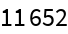### Applications

#### How much money is in your pocket (knapsack problem)?

Total given mass:

 In:=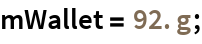Values for individual coins :

 In:=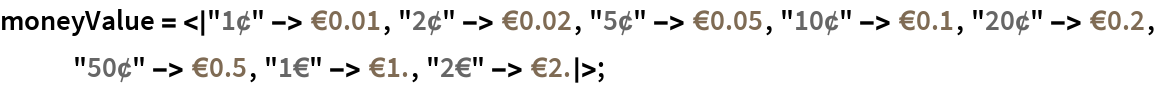In:=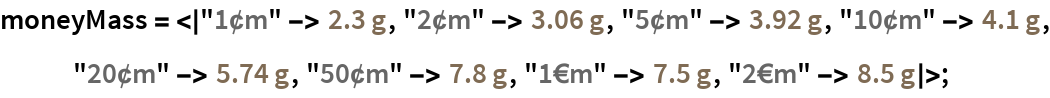Fitness function (error function). In this problem the difference between the target coin weight and the calculated coin weight is minimized:

 In:=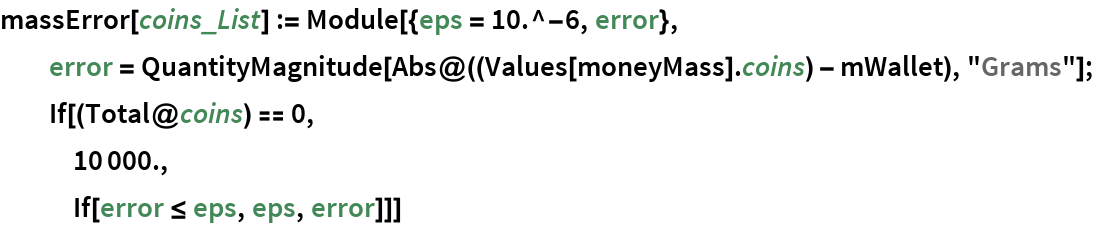Matrix of possible coins in wallet:

 In:=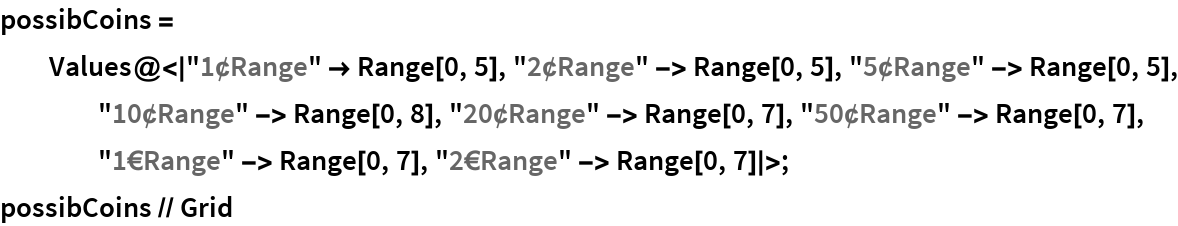Out=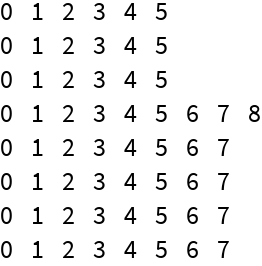Three optimization runs :

 In:=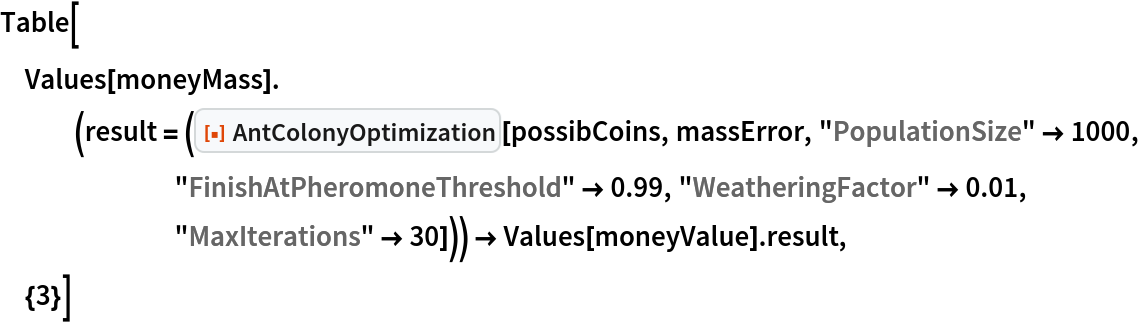Out=### Properties and Relations

Pay attention, fitness function must return a positive value. For maximization problems, the fitness must be adjusted:

 In:=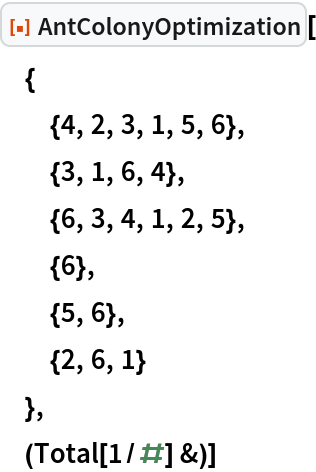Out=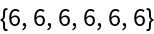### Neat Examples

#### Minimize the mass of a rod structure

Optimize a structure geometry:

 In:=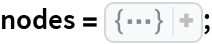In:=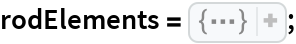In:=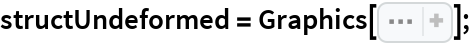In:=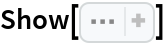Out=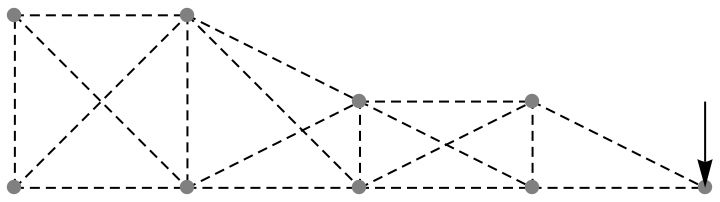In:=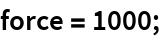Restrictions:

 In:=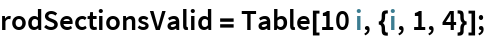In:=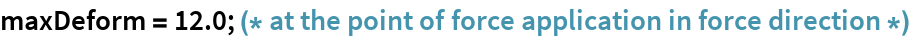In:=Out=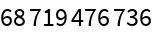Define helper functions:

 In:=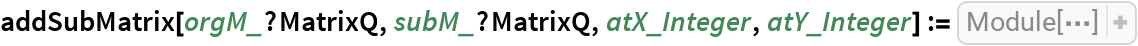In:=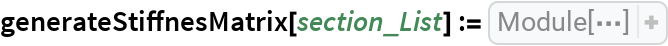In:=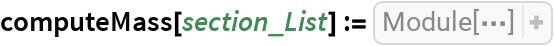In:=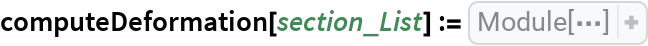The fitness function uses a penalty term to enforce constraints:

 In:=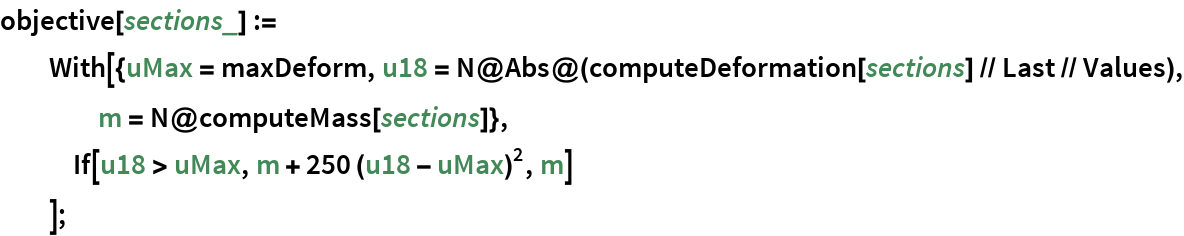Search for minimum mass structure according to the displacement constraints:

 In:=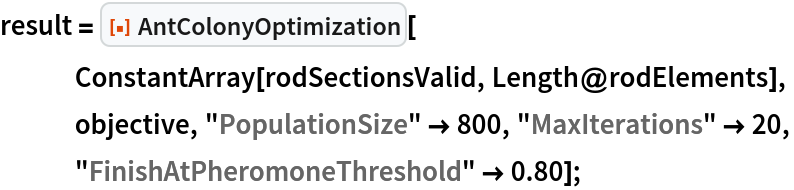Show the result :

 In:=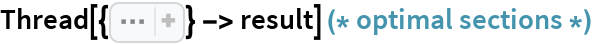Out=In:=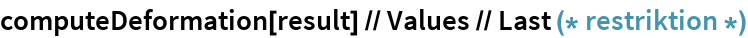Out=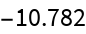In:=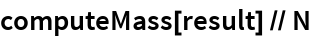Out=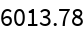Plot the result with deformed structure :

 In:=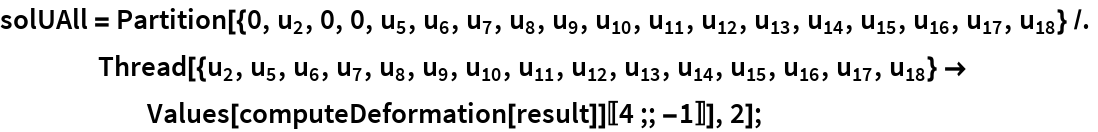In:=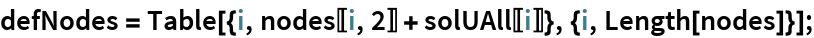In:=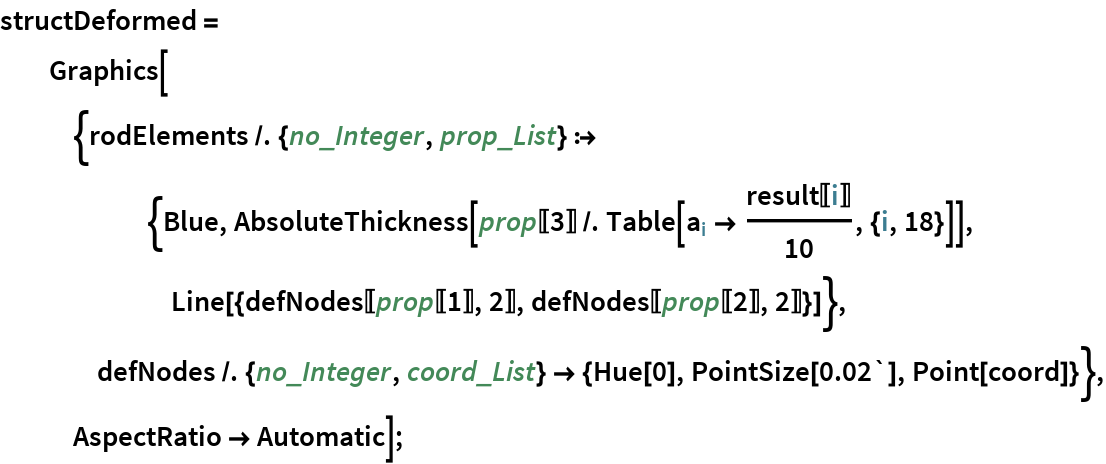In:=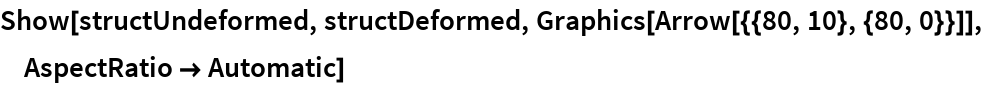Out=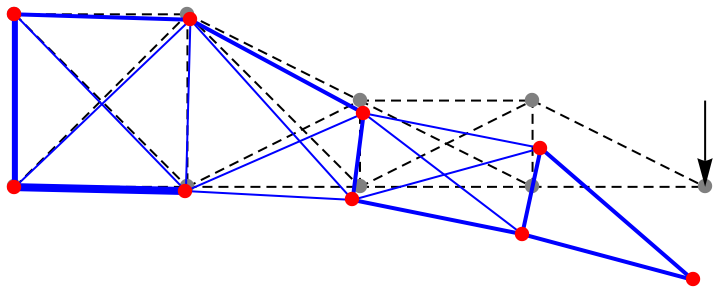## Resource History

Detailed explanation what is being optimized in this example: The array possibValues in this simple example shows the valid variable assignments in each row. Each row stands for one variable with its corresponding possible values. In this example you have 10 variables, each of them can have 10 different states. This results in 1010 possible variable assignments (size of the search space in this example).The optimization task is to find the shortest path (minimum) through this array (with its 1010 different permissible configurations) with regard to the defined function fit. For the sake of simplicity, the total value of the individual variables was simply chosen as the objective function in this example, fit=Total[#]& . Thus, for example, a path as marked in red here has a corresponding path length of Total[{3,9,1,6,10,9,7,3,6,8}]62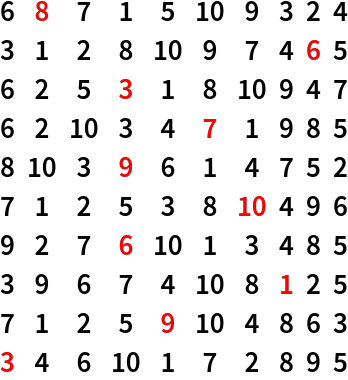The optimal (shortest possible) path to be found by the AntColonyOptimization-algorithm after some iterations is follows: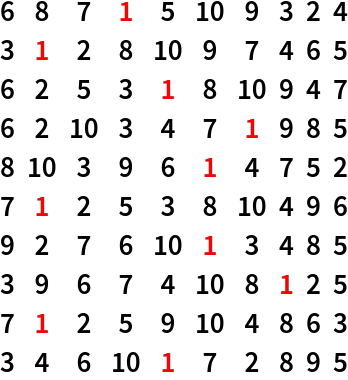with Total[{1,1,1,1,1,1,1,1,1,1}]10 (absolute minimum of the search space). This example is rather trivial and has only the purpose to outline the basic procedure and to introduce the basic parameters, such as array and fitness function, which are necessary to formulate the optimization problem. Slightly more complex application examples follow below.